Acrobat因为缺少符号，导致分析漏洞成因以及利用编写难度增加。该文章主要介绍Plugin机制和Javascript引擎，并且会直接给出相关的一些结论，这些结论主要通过官方文档和源码，再通过类比找到关键点，反复调试验证得到。

# 1. Acrobat的Plugin机制

Acrobat的SDK文档中详细介绍了Plugin机制，并且Acrobat软件本身的很多功能都是通过Plugin机制实现的（在Acrobat安装目录下的plug_ins目录下的api后缀文件，本质上是dll文件，比如支持Javascript的EScript插件，支持搜索的Search插件等）。

Acrobat主程序维护了一系列的HFT,并且在加载每一个Plugin时,会将core HFT传给Plugin，Plugin通过调用core HFT里的回调函数可以获取其他HFT（既可以是Acrobat主程序的HFT，也可以是其他Plugin注册的HFT）,并注册自己的HFT以供主程序或者其他Plugin调用，示意图如下所示。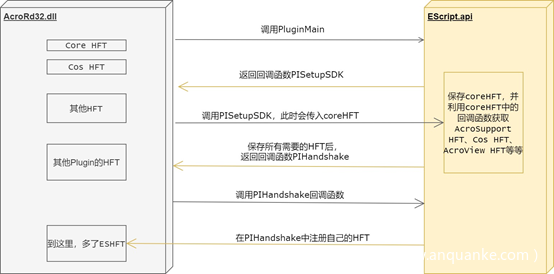## 1.1 获取coreHFT-修改对应的回调函数名称

1. Windbg调试Acrobat的主程序AcroRd32.exe(勾选Debug Child Process Also)，在调试器中断时通过sxe命令下断点（这里Plugin的名称可以自由选择，下图选择的是EScript.api）。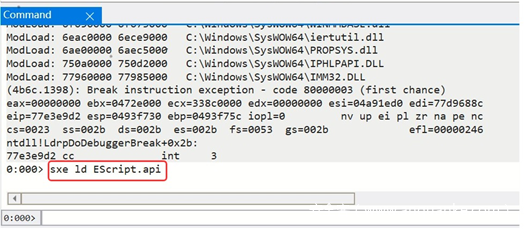1. 直接运行直到对应的Plugin加载，将IDA中通过edit->Segments->Rebase Program将IDA中的基地址和windbg中的基地址保持一致（AcroRd32.dll和其他需要的Plugin也进行同样的操作）。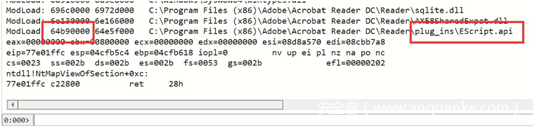1. 在IDA中阅读PluginMain函数，找到PISetupSDK回调函数，如下图所示。1. 在PISetupSDK处下断点，第2个参数是指向PISDKData_V0200的指针，PISDKData_V0200的定义如下。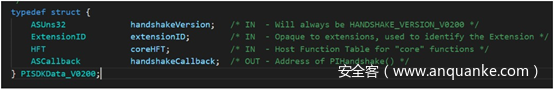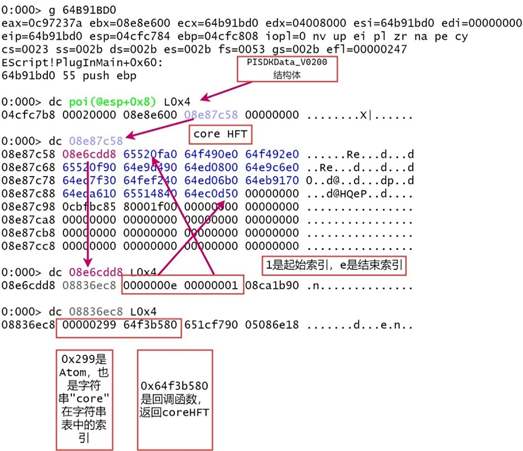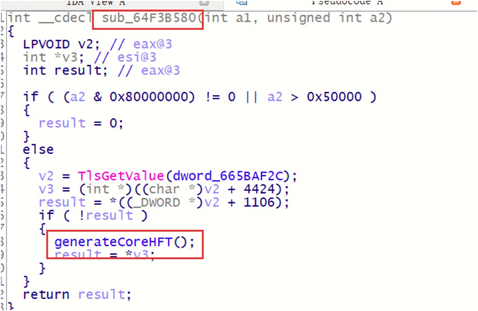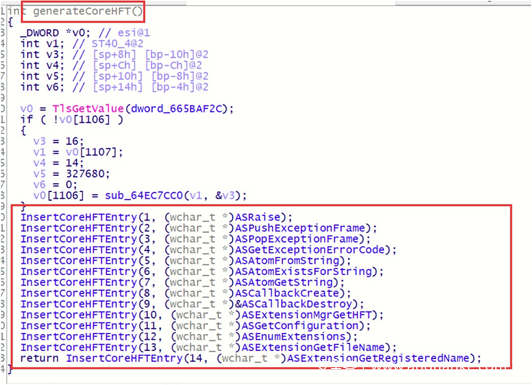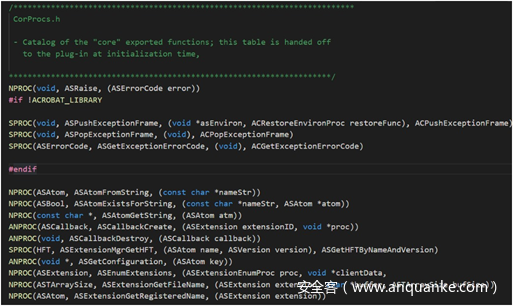## 1.2 遍历所有的Atom字符串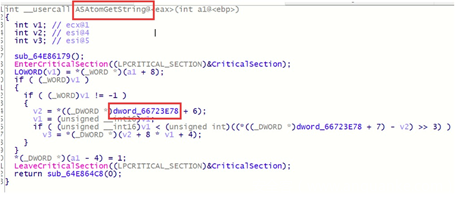``````r @\$t0 = 0x66723e78 ;

r @\$t0 = poi(@\$t0) ;

r @\$t1 = poi(@\$t0 + 0x1c) ;

r @\$t0 = poi(@\$t0 + 0x18) + 0x4 ;

.for(r @\$t2 = 0;@\$t0 + @\$t2 * 8 < @\$t1; r @\$t2 = @\$t2 + 1) {r @\$t2;r @\$t3 = @\$t0 + @\$t2 * 8;da poi(@\$t3);}``````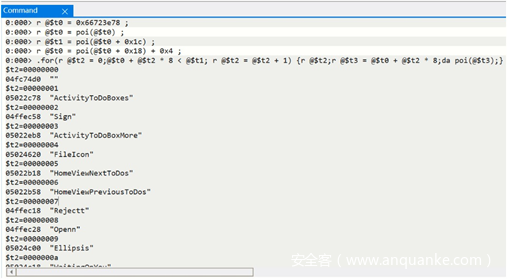``````r @\$t4 = 0x299 ;

r @\$t0 = 0x66723e78 ;

r @\$t0 = poi(@\$t0) ;

r @\$t0 = poi(@\$t0 + 0x18) + 0x4 ;

r @\$t0 = @\$t0 + @\$t4 * 8 ;

da poi(@\$t0) ;``````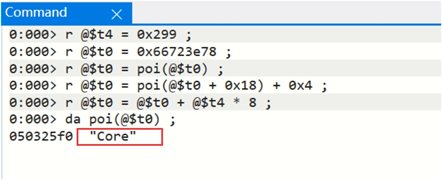## 1.3 获取所有的HFT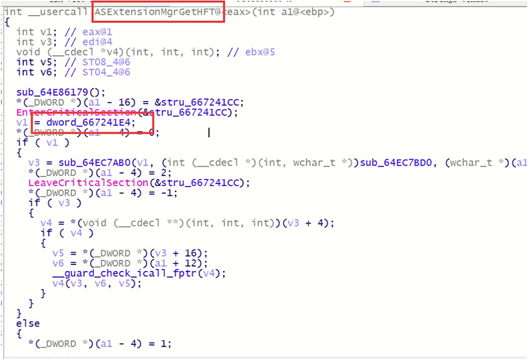``````r @\$t5 = 0x66723e78 ;

r @\$t0 = 0x667241E4;

r @\$t0 = poi(@\$t0);

r @\$t1 = poi(@\$t0) - 0x4;

r @\$t0 = poi(@\$t0 + 0xc);

r @\$t5 = poi(@\$t5) ;

r @\$t5 = poi(@\$t5 + 0x18) + 0x4 ;

.for(r @\$t2 = 0; @\$t2 <= @\$t1; r @\$t2 = @\$t2 + 1) {r @\$t3 = poi(@\$t0 + @\$t2 * 4);dd @\$t3 L0x2;r @\$t4 = low(poi(@\$t3));r @\$t6 = @\$t5 + @\$t4 * 8 ;da poi(@\$t6) ;.echo ------------------------------------}``````

``````0:000> r @\$t5 = 0x66723e78 ;

0:000> r @\$t0 = 0x667241E4;

0:000> r @\$t0 = poi(@\$t0);

0:000> r @\$t1 = poi(@\$t0) - 0x4;

0:000> r @\$t0 = poi(@\$t0 + 0xc);

0:000> r @\$t5 = poi(@\$t5) ;

0:000> r @\$t5 = poi(@\$t5 + 0x18) + 0x4 ;

0:000> .for(r @\$t2 = 0; @\$t2 <= @\$t1; r @\$t2 = @\$t2 + 1) {r @\$t3 = poi(@\$t0 + @\$t2 * 4);dd @\$t3 L0x2;r @\$t4 = low(poi(@\$t3));r @\$t6 = @\$t5 + @\$t4 * 8 ;da poi(@\$t6) ;.echo ------------------------------------}

08836ec8  00000299 64f3b580

050325f0  "Core"

------------------------------------

08836ce8  0000029a 64f3b8e0

05033248  "AcroSupport"

------------------------------------

08836c20  00001048 65527f40

08836ae0  "ASExternalWarningHandler"

------------------------------------

08836b58  00001049 00000000

05025940  "ASTest"

------------------------------------

0885e128  0000104b 00000000

------------------------------------

0885e4e8  0000029b 64f3bb80

05032670  "Cos"

------------------------------------

------------------------------------未显示完``````

1.3.1 Cos HFT

``````------------------------------------

0885e4e8  0000029b 64f3bb80

05032670  "Cos"

------------------------------------``````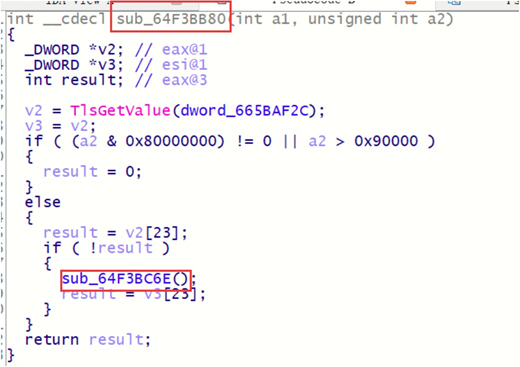``````int sub_64F3BC6E()

{

_DWORD *v0; // esi@1

int v1; // ST40_4@2

int v3; // [sp+8h] [bp-10h]@2

int v4; // [sp+Ch] [bp-Ch]@2

int v5; // [sp+10h] [bp-8h]@2

int v6; // [sp+14h] [bp-4h]@2

v0 = TlsGetValue(dword_665BAF2C);

if ( !v0 )

{

v3 = 16;

v1 = v0;

v4 = 109;

v5 = 589824;

v6 = 0;

v0 = sub_64EC7CC0(v1, &v3);

}

sub_64F3C33F(1, sub_64F82ED0, 0);

sub_64F3C33F(2, sub_64F13BA0, 0);

sub_64F3C33F(3, sub_64F97C60, 0);

sub_64F3C33F(4, sub_64F81580, 0);

-----------未 显 示 完

return sub_64F3C33F(109, sub_6536C480, 0);

}``````

``````NPROC(ASBool, CosObjEqual, (CosObj obj1, CosObj obj2))

NPROC(CosType, CosObjGetType, (CosObj obj))

NPROC(ASBool, CosObjIsIndirect, (CosObj obj))

NPROC(ASBool, CosObjEnum, (CosObj obj, CosObjEnumProc proc, void *clientData))

NPROC(CosDoc, CosObjGetDoc, (CosObj obj))

NPROC(CosObj, CosNewNull, (void))

NPROC(CosObj, CosNewInteger, (CosDoc dP, ASBool indirect, ASInt32 value))

NPROC(CosObj, CosNewFixed, (CosDoc dP, ASBool indirect, ASFixed value))

NPROC(CosObj, CosNewBoolean, (CosDoc dP, ASBool indirect, ASBool value))

NPROC(CosObj, CosNewName, (CosDoc dP, ASBool indirect, ASAtom name))

NPROC(CosObj, CosNewString, (CosDoc dP, ASBool indirect, const char *str, ASTArraySize nBytes))

NPROC(CosObj, CosNewArray, (CosDoc dP, ASBool indirect, ASTArraySize nElements))

---------未 完``````

``````------------------------------------

08899e18  00001060 64f3cf70

------------------------------------``````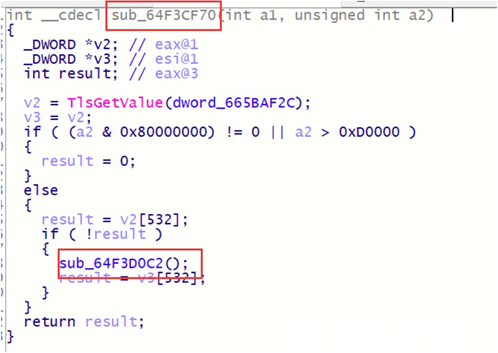``````int sub_64F3D0C2()

{

int v0; // ebp@0

_DWORD *v1; // esi@1

int v2; // ST40_4@2

int v4; // [sp+8h] [bp-10h]@2

int v5; // [sp+Ch] [bp-Ch]@2

int v6; // [sp+10h] [bp-8h]@2

int v7; // [sp+14h] [bp-4h]@2

v1 = TlsGetValue(dword_665BAF2C);

if ( !v1 )

{

v4 = 16;

v2 = v1;

v5 = 53;

v6 = 851968;

v7 = 0;

v1 = sub_64EC7CC0(v0);

}

sub_64F3D3FD(1, sub_64FC8F90, 0);

sub_64F3D3FD(2, sub_64FC9120, 0);

sub_64F3D3FD(3, sub_64FC9480, 0);

sub_64F3D3FD(4, sub_64FE89D0, 0);

sub_64F3D3FD(5, sub_654BD830, 0);

sub_64F3D3FD(6, sub_654BD890, 0);

--------------------------------

sub_64F3D3FD(52, sub_654BE790, 0);

return sub_64F3D3FD(53, sub_654BF1F0, 0);

}``````

``````NPROC (ASBool,  PDDocGetStructTreeRoot,     (IN  PDDoc pdDoc,

NPROC (ASInt32, PDSTreeRootGetNumKids,      (IN  PDSTreeRoot treeRoot))

NPROC (void,    PDSTreeRootGetKid,          (IN  PDSTreeRoot treeRoot,

NPROC (ASBool,  PDSTreeRootGetRoleMap,      (IN  PDSTreeRoot treeRoot,

NPROC (ASBool,  PDSTreeRootGetClassMap,     (IN  PDSTreeRoot treeRoot,

-------------------------------------``````

1.3.3 Forms HFT

``````------------------------------------

0889f408  006310aa 64f46f90

088d0cb0  "Forms"

------------------------------------``````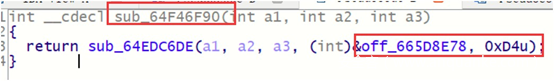``````.data:665D8E78 off_665D8E78    dd offset sub_65785D30  ; DATA XREF: sub_64F46F90+8o

.data:665D8E7C                 dd offset sub_6577D150

.data:665D8E80                 dd offset sub_6577CF80

.data:665D8E84                 dd offset sub_6577D090

.data:665D8F44                 dd offset sub_6577D4A0

-----------------------------------------------------

.data:665D8F48                 dd offset sub_6577C9D0

.data:665D8F4C                 align 10h

.data:665D8F50 off_665D8F50    dd offset sub_6577CB10  ; DATA XREF: sub_64F46FF0+8o``````

``````PIPROC(ASBool, IsPDDocAcroForm, (PDDoc doc), doc)

PIPROC(void, AFPDDocEnumPDFields, (PDDoc doc, ASBool terminals, ASBool parameterIgnored, AFPDFieldEnumProc proc, void *clientData),doc, terminals, parameterIgnored, proc, clientData)

-------------------------------------------------``````

1.3.4 EScript HFT

EScript HFT并没有包含在Acrobat SDK中，也就是无法重命名回调函数。

``````------------------------------------

0889f890  000010b5 64edc6b0

088d0f00  "ESHFT"

------------------------------------

0889f7a0  000010b6 64edcdc0

------------------------------------

0889f9a8  000010b7 64f47240

------------------------------------

0889fbd8  000010be 6515b270

088cf710  "EFSInfo"

0889fde0  000010c1 64f82950

088cf920  "Updater"

------------------------------------

0889fd68  000010c2 64f51680

088eaff8  "PrivPubSecHFT"

------------------------------------

08d7ae98  04f911e7 64b921a0

0888beb8  "\$ESHFT"``````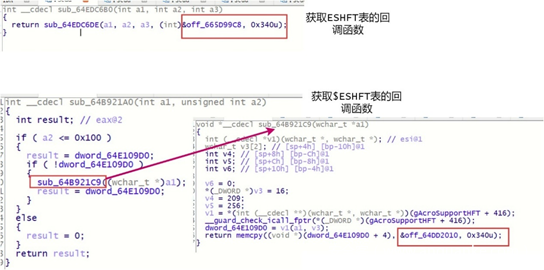1.3.5 Search HFT

1. 首先在Plugin的导出函数PluginMain中得到PISetupSDK函数。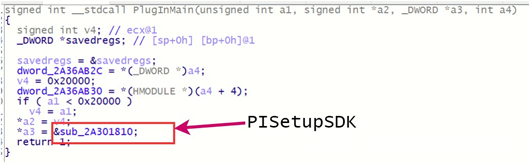1. 然后再PISetupSDK函数中得到handShake函数。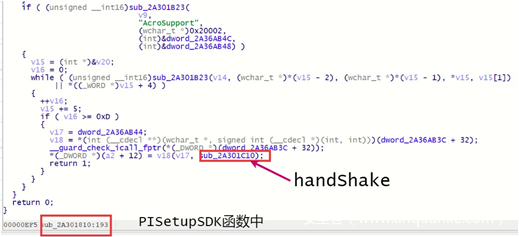1. 在handShake中，找到导出HFT的代码。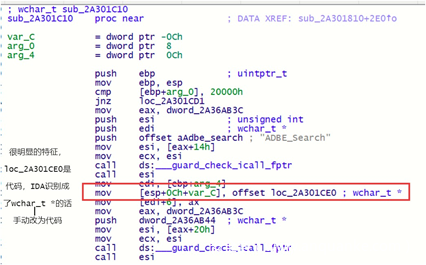4）导出HFT的代码不同插件实现可能不同，关键就是在汇编层面找”push 函数地址”这样的形式，针对这样形式的push指令，每一个函数地址都跟进去看一下，经验足够的话是很容易判断出最终的HFT表的。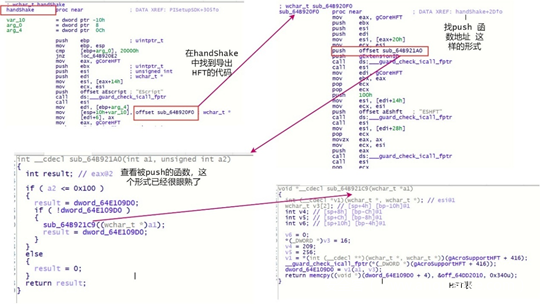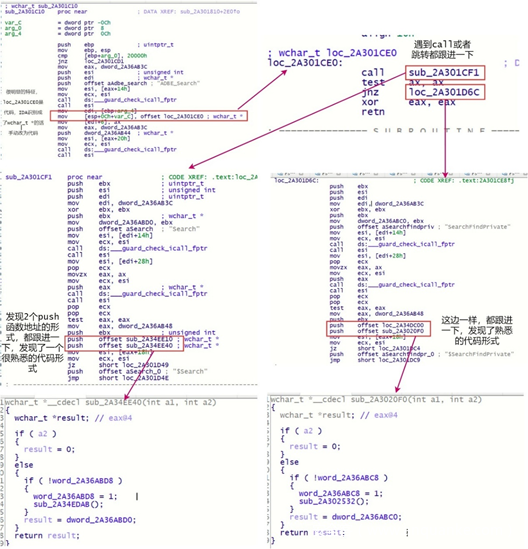## 1.4 结束语

Acrobat core API的架构如下。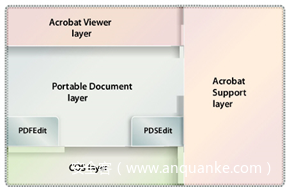Acrobat Viewer —————— Acrobat SDK中以AV为前缀的文件，HFT在AVProcs.h中声明

Acrobat Support —————— Acrobat SDK中以AS为前缀的文件，HFT在ASProcs.h中声明

COS —————— Acrobat SDK中以Cos为前缀的文件，HFT在CosProcs.h中声明

Portable Document —————— Acrobat SDK中以PD为前缀的文件，HFT在PDProcs.h中声明

# 2. Acrobat的Javascript机制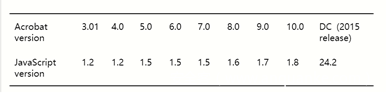SpiderMonkey 24.2是一个稳定发行版本，源码可以在http://ftp.mozilla.org/pub/spidermonkey/releases/下载。

## 2.1 查找Javascript层的api对应的Native实现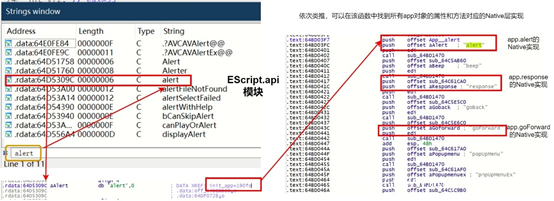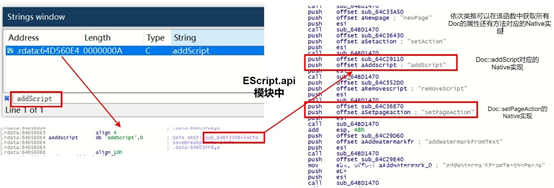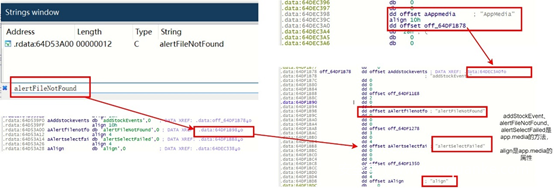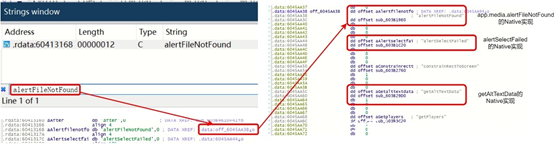## 2.2 SpiderMonkey关键结构

2.2.1 Value结构体

Javascript是无类型语言，但是这只是语言层面而言，在底层一定是要有和类型相关的信息的，Value结构体的功能就是如此（可以参考vbs的variant类型）。

``````JS_ENUM_HEADER(JSValueType, uint8_t)

{

JSVAL_TYPE_DOUBLE              = 0x00,

JSVAL_TYPE_INT32               = 0x01,

JSVAL_TYPE_UNDEFINED           = 0x02,

JSVAL_TYPE_BOOLEAN             = 0x03,

JSVAL_TYPE_MAGIC               = 0x04,

JSVAL_TYPE_STRING              = 0x05,

JSVAL_TYPE_NULL                = 0x06,

JSVAL_TYPE_OBJECT              = 0x07,

/* These never appear in a jsval; they are only provided as an out-of-band value. */

JSVAL_TYPE_UNKNOWN             = 0x20,

JSVAL_TYPE_MISSING             = 0x21

} JS_ENUM_FOOTER(JSValueType);``````

``````this["0"] = 0x5

this["1"] = 0x100000000

this["2"] = 3.14

this["3"] = undefined

this["4"] = false

this["5"] = true

this["6"] = null

this["7"] = "str1"

this["8"] = {}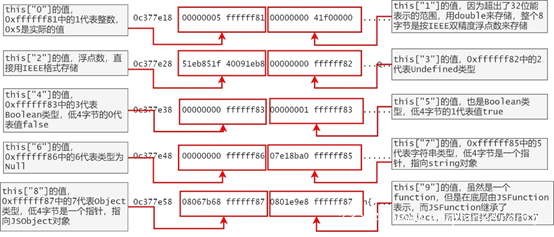2.2.2 String对象

String对象的第1个4字节由字符串字符长度（不是字节长度）和flag组成（低4位用于保存flag，其余位用于保存字符串字符长度）。

``````     *   Rope         0000       0000

*   Linear       -         !0000

*   HasBase      -          xxx1

*   Dependent    0001       0001

*   Flat         -          isLinear && !isDependent

*   Undepended   0011       0011

*   Extensible   0010       0010

*   Inline       0100       isFlat && !isExtensible && (u1.chars == inlineStorage) || isInt32)

*   Stable       0100       isFlat && !isExtensible && (u1.chars != inlineStorage)

*   Short        0100       header in FINALIZE_SHORT_STRING arena

*   External     0100       header in FINALIZE_EXTERNAL_STRING arena

*   Int32        0110       x110 (NYI, Bug 654190)

*   Atom         1000       1xxx

*   InlineAtom   1000       1000 && is Inline

*   ShortAtom    1000       1000 && is Short

Int32Atom    1110       1110 (NYI, Bug 654190)``````

``this["7"] = "str1"``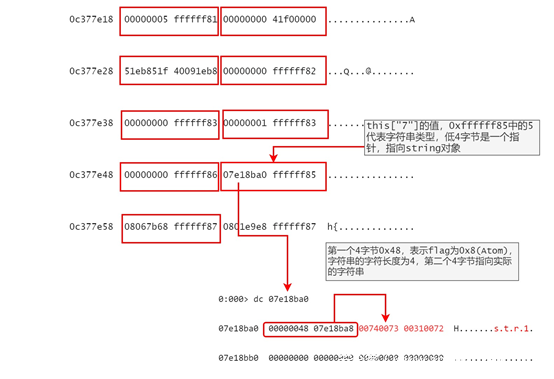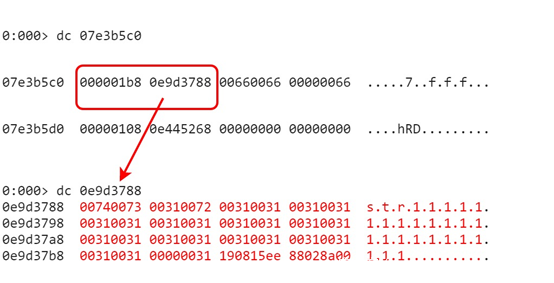2.2.3 JSObject

JSObject对应的是Javascript层的Object概念。

2.2.3.1 空Object对应的JSObject

``````this.dummy1 = 255

this.emptyObject = {}

this.dummy2 = 255``````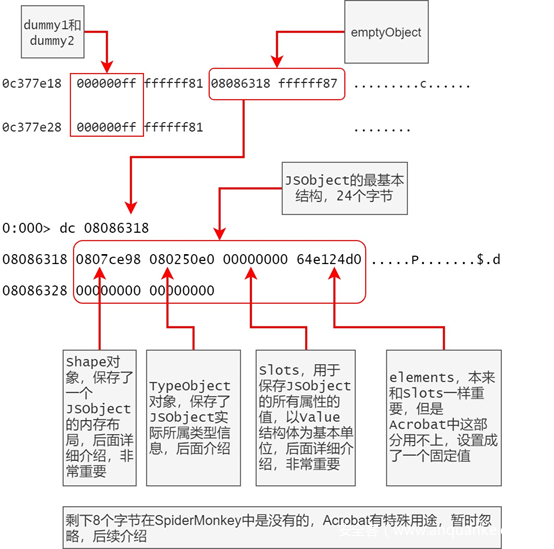2.2.3.2 Shape对象

Shape对象就是这个中间的媒介，Shape的逻辑如下图所示。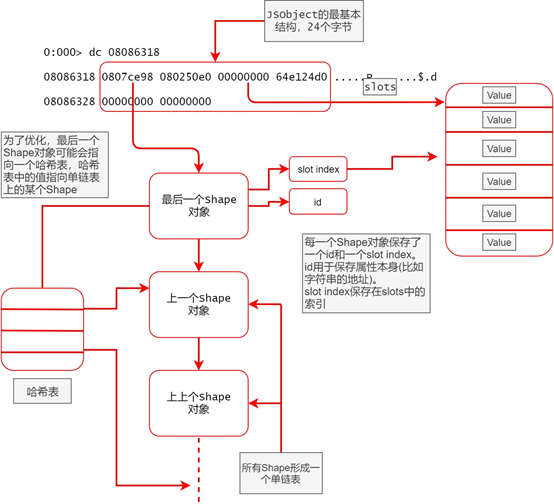① 每一个Shape对象保存了id和slot索引，通过对比id来进行所谓的查找属性，id一致后就可以根据对应的slot索引得到属性的值。

② 一个JSObject的第一个成员指向Shape单链表的最后一个元素。

③ 单链表的最后一个Shape对象可能会指向一个哈希表。

④ 查找属性的时候，先通过id尝试在哈希表里直接获取对应的Shape（如果有哈希表的话）。

⑤ 如果没有找到或者哈希表不存在，则通过遍历单链表，然后对比id来查找Shape。

⑥ 如果找到了Shape，则根据Shape中的slot索引获取属性的值。

``````this.dummy1 = 255

this.emptyObject = {}

this.dummy2 = 255

this.emptyObject.mem1 = "mem1"

this.emptyObject.mem2 = "mem2"

this.emptyObject.mem3 = "mem3"

this.emptyObject.mem4 = "mem4"

this.emptyObject.mem5 = "mem5"``````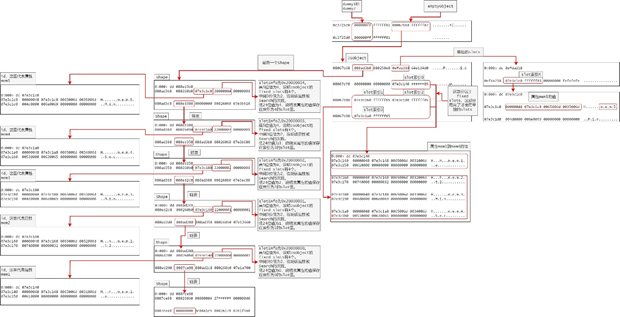``````r @\$t0 = 0x08067b68 ;

r @\$t1 = poi(@\$t0) ;

r @\$t2 = poi(@\$t0 + 0x8 );

r @\$t8 = poi(@\$t1 + 0x10);

.for(; @\$t8 != 0; r @\$t1 = @\$t8;r @\$t8 = poi(@\$t1 + 0x10)) {r @\$t3 = poi(@\$t1 + 0x4);r @\$t9 = 0;.catch {du poi(@\$t3 + 0x4); r @\$t9 = 0xaa};.if (@\$t9 != 0xaa) {r @\$t3};r @\$t3 = poi(@\$t1 + 0x8);r @\$t4 = @\$t3 >> 0n27;r @\$t5 = @\$t3 & 0x00ffffff;r @\$t4, @\$t5;.if(@\$t5 < @\$t4) {dd @\$t0+0x18+@\$t5*8 L0x2;}.else {r @\$t5 = @\$t5-@\$t4;dd @\$t2+@\$t5*8 L0x2;};.echo --------------------;}``````

``````0:000> r @\$t0 = 0x08067b68 ;

0:000> r @\$t1 = poi(@\$t0) ;

0:000> r @\$t2 = poi(@\$t0 + 0x8 );

0:000> r @\$t8 = poi(@\$t1 + 0x10);

0:000> .for(; @\$t8 != 0; r @\$t1 = @\$t8;r @\$t8 = poi(@\$t1 + 0x10)) {r @\$t3 = poi(@\$t1 + 0x4);r @\$t9 = 0;.catch {du poi(@\$t3 + 0x4); r @\$t9 = 0xaa};.if (@\$t9 != 0xaa) {r @\$t3};r @\$t3 = poi(@\$t1 + 0x8);r @\$t4 = @\$t3 >> 0n27;r @\$t5 = @\$t3 & 0x00ffffff;r @\$t4, @\$t5;.if(@\$t5 < @\$t4) {dd @\$t0+0x18+@\$t5*8 L0x2;}.else {r @\$t5 = @\$t5-@\$t4;dd @\$t2+@\$t5*8 L0x2;};.echo --------------------;}

07e3c1c8  "mem5"

\$t4=00000004 \$t5=00000004

0efda218  07e3c1c0 ffffff85

--------------------

07e3c1a8  "mem4"

\$t4=00000004 \$t5=00000003

08067b98  07e3c1a0 ffffff85

--------------------

07e3c188  "mem3"

\$t4=00000004 \$t5=00000002

08067b90  07e3c180 ffffff85

--------------------

07e3c168  "mem2"

\$t4=00000004 \$t5=00000001

08067b88  07e3c160 ffffff85

--------------------

07e3c148  "mem1"

\$t4=00000004 \$t5=00000000

08067b80  07e3c140 ffffff85

--------------------``````

2.2.3.3 TypeObject对象

TypeObject有2个关键成员，第1个是clasp——可以用来判断一个Object所属类型；第2个是proto——Javascript中的prototype概念的实现。

proto在查找对象属性的时候会用到，如果当前JSObject的Shape单链表中没有找到属性，则会在proto的Shape单链表继续查找，依次类推。

``````this.dummy1 = 255

this.proto = {}

this.emptyObject = {}

this.dummy2 = 255

this.proto.mem1 = "proto"

this.emptyObject.__proto__ = this.proto``````

TypeObject演示如下。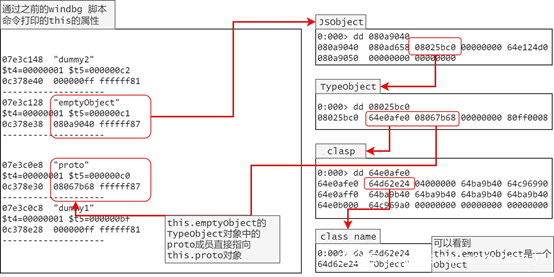2.2.4 JSFunction和JSScript

JSFunction对应的是Javascript层function的概念。

``````this.dummy1 = 255

this.dummy2 = 255``````

JSFunction的逻辑如下所示。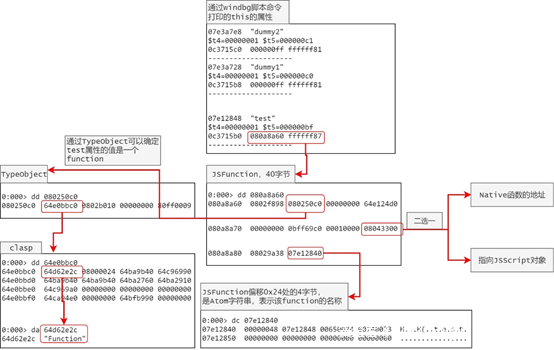0x24处的4字节指向一个Atom字符串，该字符串表示function的名称，注意这里的”test”和打印this的属性时的字符串”test”含义是不一样的，打印this的属性时的字符串”test”表示的是this对象的属性名称，这个属性不一定是”test”，但是function的名称确定后就不会变了。

0x1c处的4字节要么是一个Native函数的地址（如果JSFunction封装的是Native函数的话），要么指向一个JSScript对象(调用函数的话相应的字节码会被解释执行)。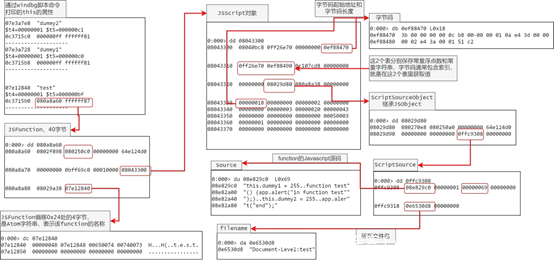ScriptSource对象包含了对应function的Javascript源码和源码所在的文件名。

JSScript可以用来在调试过程中辅助判断某个对象。

2.2.5 Array对象

Array对象是基于JSObject的，对于如下代码

``````this.dummy1 = 255

this.arr = Array(255, "elem2", 255)

this.arr.push({})

this.dummy2 = 255``````

this.arr的实际存储形式如下。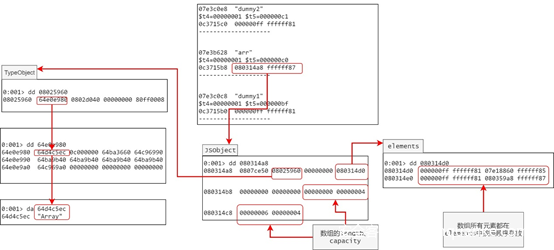## 2.2.6 Map对象

Map对象也是基于JSObject，不过涉及到Hash，所以稍微复杂一些。

``````this.dummy1 = 255

this.map = new Map()

this.map.set("key1", "value1")

this.map.set("key2", "value2")

this.map.set("key3", "value3")

this.dummy2 = 255``````

this.map的实际存储形式如下。2.2.7 Set对象

Set对象和Map对象很类似，而且都是基于Ordered HashTable。

``````this.dummy1 = 255

this.set = new Set()

this.dummy2 = 255``````

this.set的实际存储形式如下。2.2.8 FrameRegs和StackFrame

FrameRegs和StackFrame是SpiderMonkey的解释器解释执行字节码时需要的2个最关键的结构，它们都是Interpret函数中频繁使用到的变量。

FrameRegs结构包含3个成员，依次是sp_、pc_和fp_。

sp_成员模拟esp(rsp)寄存器，pc_成员模拟eip(rip)寄存器，fp_成员模拟ebp(rbp)寄存器。

sp_永远指向栈顶，pc_一开始指向字节码的起始地址，在解释执行的过程中会指向下一个字节码，fp_指向的是一个StackFrame结构体。

StackFrame保存了当前的作用域、局部变量、当前的脚本、this、arguments和callee等关键信息。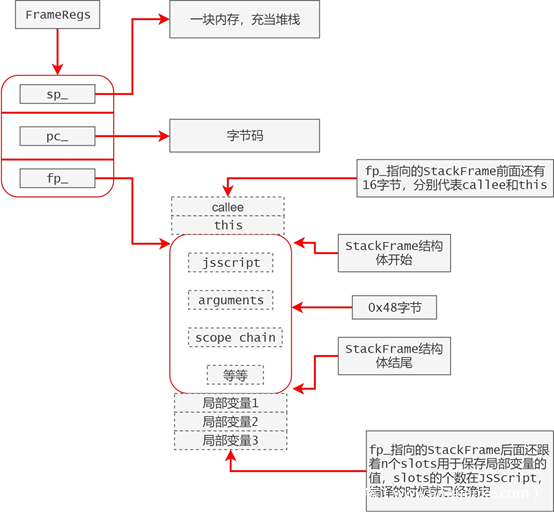## 2.3 实战

2.3.1 准备pdf

Acrobat Pro要收费，PDF-XChange-Editor可以免费使用，不过生成的pdf有水印。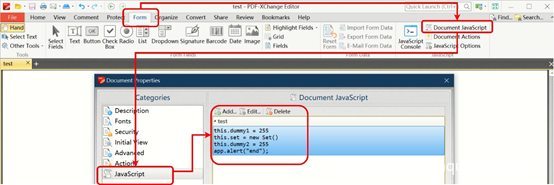crtl+break或者alt+delete强行中断到windbg，输入lmm escript查看EScript.api模块的基地址。

``````0:034> lmm escript

Browse full module list

start    end        module name

64b90000 64e5f000   EScript    (deferred)   ``````

IDA加载EScript.api，并重定向基地址为实际的地址。

2.3.3 查找Interpret——解释执行pcode(字节码)的函数

1. 直接在IDA中搜索文本“switch 230 cases”，得到的结果就在Interpret函数中。1. 在字符串窗口找到字符串”js::RunScript”，然后通过交叉引用进入到引用该字符串的函数，再通过交叉引用退回到上一层函数，该函数就是js::RunScript。2.3.4 细粒度调试Javascript代码

1. 在PDF-XChange-Editor中生成带有如下javascript代码的pdf文档。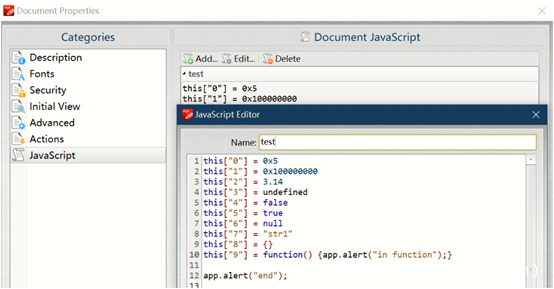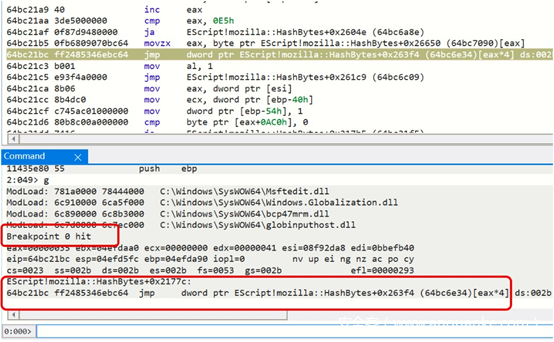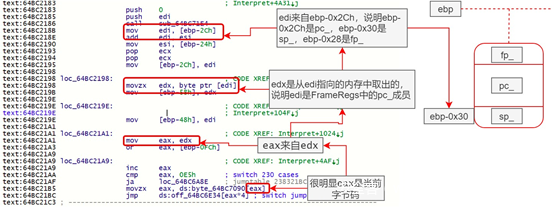1. 通过StackFrame（[ebp-0x28]）获取this指针（在这次实验中this代表的是Doc对象），如下图所示。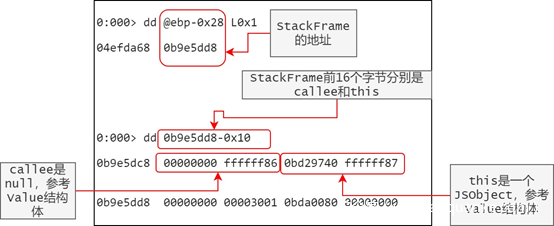1. 将值0xbd29740替换2.2.3.2节中的Windbg脚本命令中的@\$t0寄存器，运行后就可以查看this（Doc对象）当前的所有属性。

Windbg脚本命令运行结果如下所示（为了篇幅把中间大部分内容省了）。

``````0:000> r @\$t0 = 0xbd29740 ;

0:000> r @\$t1 = poi(@\$t0) ;

0:000> r @\$t2 = poi(@\$t0 + 0x8 );

0:000> r @\$t8 = poi(@\$t1 + 0x10);

0:000> .for(; @\$t8 != 0; r @\$t1 = @\$t8;r @\$t8 = poi(@\$t1 + 0x10)) {r @\$t3 = poi(@\$t1 + 0x4);r @\$t9 = 0;.catch {du poi(@\$t3 + 0x4); r @\$t9 = 0xaa};.if (@\$t9 != 0xaa) {r @\$t3};r @\$t3 = poi(@\$t1 + 0x8);r @\$t4 = @\$t3 >> 0n27;r @\$t5 = @\$t3 & 0x00ffffff;r @\$t4, @\$t5;.if(@\$t5 < @\$t4) {dd @\$t0+0x18+@\$t5*8 L0x2;}.else {r @\$t5 = @\$t5-@\$t4;dd @\$t2+@\$t5*8 L0x2;};.echo --------------------;}

0c6e1c50  "rightsManagement"

\$t4=00000001 \$t5=000000b9

0bcc8e28  00000000 ffffff82

--------------------

0b994ae8  "encryptUsingPolicy"

\$t4=00000001 \$t5=000000b8

0bcc8e20  0bd1e5d8 ffffff87

--------------------

0a8377c8  "layout"

\$t4=00000001 \$t5=00000002

0bcc8870  00000000 ffffff82

--------------------

0a815528  "info"

\$t4=00000001 \$t5=00000001

0bcc8868  00000000 ffffff82

--------------------

0a81c4a8  "hidden"

\$t4=00000001 \$t5=00000000

0bd29758  00000000 ffffff82

--------------------``````
1. 获取并保存了this后，接下来跟踪字节码的执行。① 通过[ebp-0x2C]发现当前字节码是0x41(65)，查询后发现是JSOP_THIS,windbg中直接输入g命令解释执行该字节码，准备执行下一个字节码的时候调试器又触发断点，此时通过[ebp-0x30]查看sp_指向的临时堆栈的栈顶。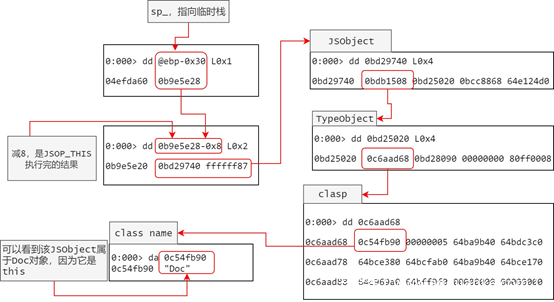② 通过[ebp-0x2C]发现当前字节码是0x3e(62)，查询后发现是JSOP_ZERO,windbg中直接输入g命令解释执行该字节码，准备执行下一个字节码的时候调试器又触发断点，此时通过[ebp-0x30]查看临时栈栈顶。

``````0:000> dd poi(@ebp-0x30)-0x8

0b9e5e28  00000000 ffffff81``````

③ 通过[ebp-0x2C]发现当前字节码是0xd7(215)，查询后发现是JSOP_INT8,windbg直接输入g命令解释执行该字节码，准备执行下一个字节码的时候调试器又触发断点，此时通过[ebp-0x30]查看临时栈栈顶。

``````0:000> dd poi(@ebp-0x30)-0x8

0b9e5e30  00000005 ffffff81``````

④ 通过[ebp-0x2C]发现当前字节码是0x38(56)，查询后发现是JSOP_SETELEM，windbg直接输入g命令执行解释该字节码，准备执行下一个字节码的时候调试器又触发断点，此时运行之前保存的打印this的所有属性的windbg脚本命令，结果如下（省略了大部分结果）。

``````0:000> r @\$t0 = 0xbd29740  ;

0:000> r @\$t1 = poi(@\$t0) ;

0:000> r @\$t2 = poi(@\$t0 + 0x8 );

0:000> r @\$t8 = poi(@\$t1 + 0x10);

0:000> .for(; @\$t8 != 0; r @\$t1 = @\$t8;r @\$t8 = poi(@\$t1 + 0x10)) {r @\$t3 = poi(@\$t1 + 0x4);r @\$t9 = 0;.catch {du poi(@\$t3 + 0x4); r @\$t9 = 0xaa};.if (@\$t9 != 0xaa) {r @\$t3};r @\$t3 = poi(@\$t1 + 0x8);r @\$t4 = @\$t3 >> 0n27;r @\$t5 = @\$t3 & 0x00ffffff;r @\$t4, @\$t5;.if(@\$t5 < @\$t4) {dd @\$t0+0x18+@\$t5*8 L0x2;}.else {r @\$t5 = @\$t5-@\$t4;dd @\$t2+@\$t5*8 L0x2;};.echo --------------------;}

Memory access error at '); r @\$t9 = 0xaa'

\$t4=00000001 \$t5=000000ba

0bcc8e30  00000005 ffffff81

--------------------

0c6e1c50  "rightsManagement"

\$t4=00000001 \$t5=000000b9

0bcc8e28  00000000 ffffff82

--------------------

0b994ae8  "encryptUsingPolicy"

\$t4=00000001 \$t5=000000b8

0bcc8e20  0bd1e5d8 ffffff87

--------------------``````

2.3.5 快速调试Javascript代码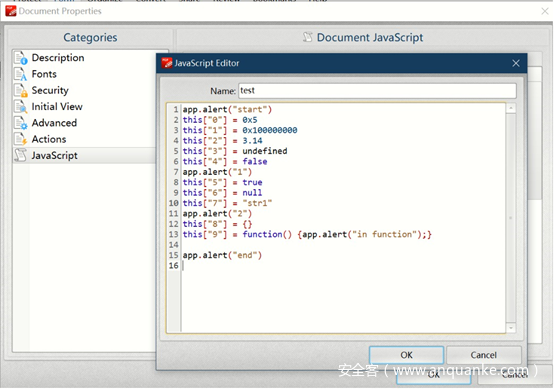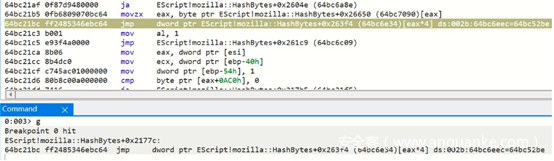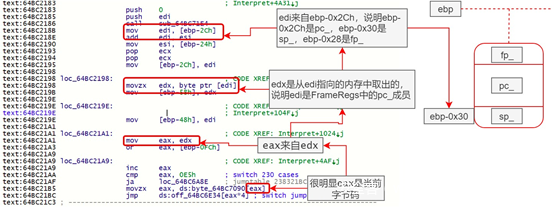1. 通过StackFrame（[ebp-0x28]）获取this指针（在这次实验中this代表的是Doc对象），如下图所示。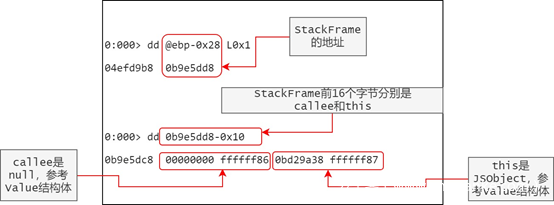1. 将值0xbd29a38替换2.2.3.2节中的Windbg脚本命令中的@\$t0寄存器，运行后就可以查看this（Doc对象）当前的所有属性。

Windbg脚本命令运行结果如下所示（为了篇幅把中间大部分内容省了）。

``````0:000> r @\$t0 = 0xbd29a38  ;

0:000> r @\$t1 = poi(@\$t0) ;

0:000> r @\$t2 = poi(@\$t0 + 0x8 );

0:000> r @\$t8 = poi(@\$t1 + 0x10);

0:000> .for(; @\$t8 != 0; r @\$t1 = @\$t8;r @\$t8 = poi(@\$t1 + 0x10)) {r @\$t3 = poi(@\$t1 + 0x4);r @\$t9 = 0;.catch {du poi(@\$t3 + 0x4); r @\$t9 = 0xaa};.if (@\$t9 != 0xaa) {r @\$t3};r @\$t3 = poi(@\$t1 + 0x8);r @\$t4 = @\$t3 >> 0n27;r @\$t5 = @\$t3 & 0x00ffffff;r @\$t4, @\$t5;.if(@\$t5 < @\$t4) {dd @\$t0+0x18+@\$t5*8 L0x2;}.else {r @\$t5 = @\$t5-@\$t4;dd @\$t2+@\$t5*8 L0x2;};.echo --------------------;}

\$t4=00000001 \$t5=000000b8

0b8ec920  0bd24ab0 ffffff87

--------------------

\$t4=00000001 \$t5=000000b7

0b8ec918  0bd24a88 ffffff87

--------------------

0a81c4a8  "hidden"

\$t4=00000001 \$t5=00000000

0bd29a50  00000000 ffffff82

--------------------``````
``````0:000> bl

0 e Disable Clear  64bc21bc     0001 (0001)  0:**** EScript!mozilla::HashBytes+0x2177c

0:000> bd 0

0:000> bl

0 d Enable Clear  64bc21bc     0001 (0001)  0:**** EScript!mozilla::HashBytes+0x2177c

0:000> g

(4b38.3950): C++ EH exception - code e06d7363 (first chance)

(4b38.3950): C++ EH exception - code e06d7363 (first chance)``````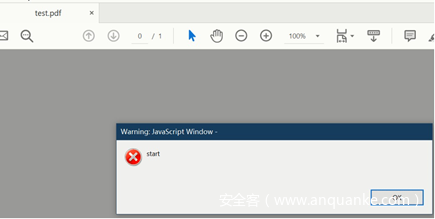1. 点击OK，弹出下一个对话框。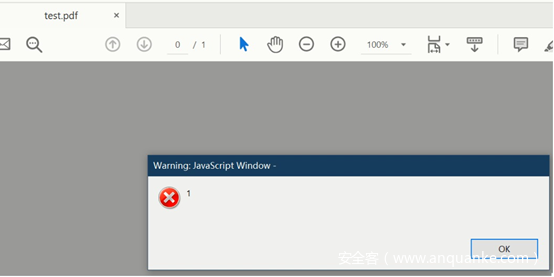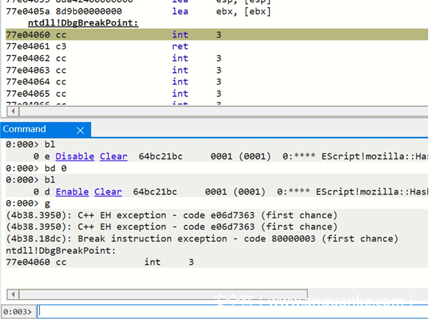1. 再次运行刚才运行过的Windbg脚本命令，结果如下。
``````0:003> r @\$t0 = 0xbd29a38  ;

0:003> r @\$t1 = poi(@\$t0) ;

0:003> r @\$t2 = poi(@\$t0 + 0x8 );

0:003> r @\$t8 = poi(@\$t1 + 0x10);

0:003> .for(; @\$t8 != 0; r @\$t1 = @\$t8;r @\$t8 = poi(@\$t1 + 0x10)) {r @\$t3 = poi(@\$t1 + 0x4);r @\$t9 = 0;.catch {du poi(@\$t3 + 0x4); r @\$t9 = 0xaa};.if (@\$t9 != 0xaa) {r @\$t3};r @\$t3 = poi(@\$t1 + 0x8);r @\$t4 = @\$t3 >> 0n27;r @\$t5 = @\$t3 & 0x00ffffff;r @\$t4, @\$t5;.if(@\$t5 < @\$t4) {dd @\$t0+0x18+@\$t5*8 L0x2;}.else {r @\$t5 = @\$t5-@\$t4;dd @\$t2+@\$t5*8 L0x2;};.echo --------------------;}

Memory access error at '); r @\$t9 = 0xaa'

\$t3=00000009

\$t4=00000001 \$t5=000000bd

0b8ec948  00000000 ffffff83

--------------------

Memory access error at '); r @\$t9 = 0xaa'

\$t3=00000007

\$t4=00000001 \$t5=000000bc

0b8ec940  00000000 ffffff82

--------------------

Memory access error at '); r @\$t9 = 0xaa'

\$t3=00000005

\$t4=00000001 \$t5=000000bb

0b8ec938  51eb851f 40091eb8

--------------------

Memory access error at '); r @\$t9 = 0xaa'

\$t3=00000003

\$t4=00000001 \$t5=000000ba

0b8ec930  00000000 41f00000

--------------------

Memory access error at '); r @\$t9 = 0xaa'

\$t3=00000001

\$t4=00000001 \$t5=000000b9

0b8ec928  00000005 ffffff81

--------------------

\$t4=00000001 \$t5=000000b8

0b8ec920  0bd24ab0 ffffff87

--------------------``````

1. 输入命令g运行，再次点击对话框的OK，弹出下一个对话框。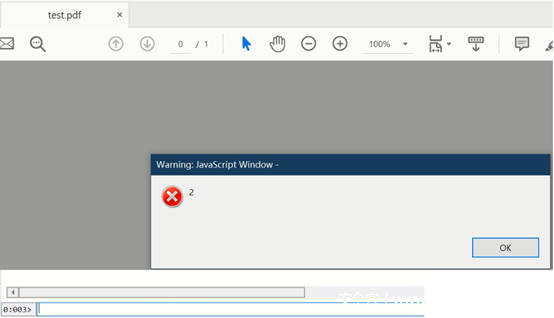1. 再次强行中断并运行Windbg脚本命令，结果如下。
``````0:001> r @\$t0 = 0xbd29a38  ;

0:001> r @\$t1 = poi(@\$t0) ;

0:001> r @\$t2 = poi(@\$t0 + 0x8 );

0:001> r @\$t8 = poi(@\$t1 + 0x10);

0:001> .for(; @\$t8 != 0; r @\$t1 = @\$t8;r @\$t8 = poi(@\$t1 + 0x10)) {r @\$t3 = poi(@\$t1 + 0x4);r @\$t9 = 0;.catch {du poi(@\$t3 + 0x4); r @\$t9 = 0xaa};.if (@\$t9 != 0xaa) {r @\$t3};r @\$t3 = poi(@\$t1 + 0x8);r @\$t4 = @\$t3 >> 0n27;r @\$t5 = @\$t3 & 0x00ffffff;r @\$t4, @\$t5;.if(@\$t5 < @\$t4) {dd @\$t0+0x18+@\$t5*8 L0x2;}.else {r @\$t5 = @\$t5-@\$t4;dd @\$t2+@\$t5*8 L0x2;};.echo --------------------;}

Memory access error at '); r @\$t9 = 0xaa'

\$t3=0000000f

\$t4=00000001 \$t5=000000c0

0b8ec960  0a818ba0 ffffff85

--------------------

Memory access error at '); r @\$t9 = 0xaa'

\$t3=0000000d

\$t4=00000001 \$t5=000000bf

0b8ec958  00000000 ffffff86

--------------------

Memory access error at '); r @\$t9 = 0xaa'

\$t3=0000000b

\$t4=00000001 \$t5=000000be

0b8ec950  00000001 ffffff83

--------------------

Memory access error at '); r @\$t9 = 0xaa'

\$t3=00000009

\$t4=00000001 \$t5=000000bd

0b8ec948  00000000 ffffff83

--------------------

Memory access error at '); r @\$t9 = 0xaa'

\$t3=00000007

\$t4=00000001 \$t5=000000bc

0b8ec940  00000000 ffffff82

--------------------

Memory access error at '); r @\$t9 = 0xaa'

\$t3=00000005

\$t4=00000001 \$t5=000000bb

0b8ec938  51eb851f 40091eb8

--------------------

Memory access error at '); r @\$t9 = 0xaa'

\$t3=00000003

\$t4=00000001 \$t5=000000ba

0b8ec930  00000000 41f00000

--------------------

Memory access error at '); r @\$t9 = 0xaa'

\$t3=00000001

\$t4=00000001 \$t5=000000b9

0b8ec928  00000005 ffffff81

--------------------

\$t4=00000001 \$t5=000000b8

0b8ec920  0bd24ab0 ffffff87

--------------------``````

1. 再次输入g运行，点击对话框的OK，弹出下一个对话框。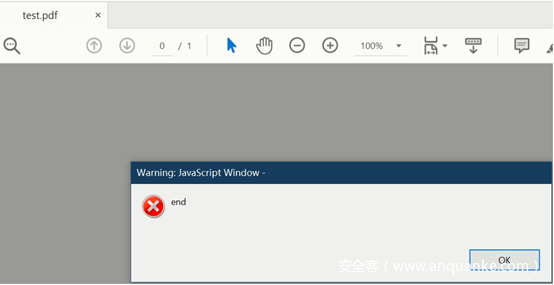1. 再次强行中断并运行Windbg脚本命令，结果如下。
``````0:003> r @\$t0 = 0xbd29a38  ;

0:003> r @\$t1 = poi(@\$t0) ;

0:003> r @\$t2 = poi(@\$t0 + 0x8 );

0:003> r @\$t8 = poi(@\$t1 + 0x10);

0:003> .for(; @\$t8 != 0; r @\$t1 = @\$t8;r @\$t8 = poi(@\$t1 + 0x10)) {r @\$t3 = poi(@\$t1 + 0x4);r @\$t9 = 0;.catch {du poi(@\$t3 + 0x4); r @\$t9 = 0xaa};.if (@\$t9 != 0xaa) {r @\$t3};r @\$t3 = poi(@\$t1 + 0x8);r @\$t4 = @\$t3 >> 0n27;r @\$t5 = @\$t3 & 0x00ffffff;r @\$t4, @\$t5;.if(@\$t5 < @\$t4) {dd @\$t0+0x18+@\$t5*8 L0x2;}.else {r @\$t5 = @\$t5-@\$t4;dd @\$t2+@\$t5*8 L0x2;};.echo --------------------;}

Memory access error at '); r @\$t9 = 0xaa'

\$t3=00000013

\$t4=00000001 \$t5=000000c2

0b8ec970  0bd24b50 ffffff87

--------------------

Memory access error at '); r @\$t9 = 0xaa'

\$t3=00000011

\$t4=00000001 \$t5=000000c1

0b8ec968  0bda9078 ffffff87

--------------------

Memory access error at '); r @\$t9 = 0xaa'

\$t3=0000000f

\$t4=00000001 \$t5=000000c0

0b8ec960  0a818ba0 ffffff85

--------------------``````

2.3.6 调用Native function的逻辑

2.2.4已经介绍了JSFunction的概念，这里主要通过实际调试直观地感受一下之前的概念。

1. 生成一个包含如下代码的pdf。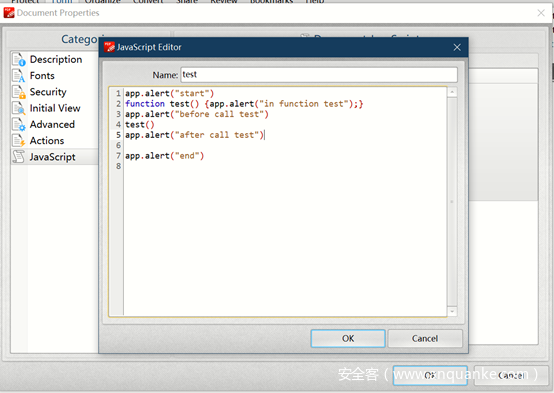1. 根据之前的技巧调试该pdf，触发断点，找到this的值为0x0bd29a38。

2. 使用windbg脚本命令打印this的所有属性，找到app属性及对应的值。

``````--------------------

0a819ce8  "app"

\$t4=00000001 \$t5=00000058

0b8ebe18  0bd29b78 ffffff87

--------------------``````
1. 可以看到app对象的地址是0x0bd29b78，再次使用windbg脚本命令打印app对象的所有属性。
``````0:000> r @\$t0 = 0x0bd29b78  ;

0:000> r @\$t1 = poi(@\$t0) ;

0:000> r @\$t2 = poi(@\$t0 + 0x8 );

0:000> r @\$t8 = poi(@\$t1 + 0x10);

0:000> .for(; @\$t8 != 0; r @\$t1 = @\$t8;r @\$t8 = poi(@\$t1 + 0x10)) {r @\$t3 = poi(@\$t1 + 0x4);r @\$t9 = 0;.catch {du poi(@\$t3 + 0x4); r @\$t9 = 0xaa};.if (@\$t9 != 0xaa) {r @\$t3};r @\$t3 = poi(@\$t1 + 0x8);r @\$t4 = @\$t3 >> 0n27;r @\$t5 = @\$t3 & 0x00ffffff;r @\$t4, @\$t5;.if(@\$t5 < @\$t4) {dd @\$t0+0x18+@\$t5*8 L0x2;}.else {r @\$t5 = @\$t5-@\$t4;dd @\$t2+@\$t5*8 L0x2;};.echo --------------------;}

0a81e7c8  "doc"

\$t4=00000001 \$t5=00000000

0bd29b90  0bd29a38 ffffff87

--------------------``````

1. 根据2.2.3.3得到app对象的prototype。
``````0:000> dd 0x0bd29b78 L0x4

0bd29b78  0bdb5df0 0bd25980 00000000 64e124d0

0:000> dd 0bd25980 L0x4

0bd25980  0b9f7110 0bd29268 00000000 80ff0008``````

``````--------------------

\$t4=00000001 \$t5=0000000d

0b99b040  0bd3c3d0 ffffff87

--------------------``````

``````0:000> dd 0xbd3c3d0 L0xa

0bd3c3d0  0bd3b8c8 0bd250c0 00000000 64e124d0

0bd3c3e0  0c763b00 00000000 00000000 64be4600

0bd3c3f0  00000000 0a818cc0

0:000> dc 0a818cc0 L0x8

0a818cc0  00000058 0a818cc8 006c0061 00720065  X.......a.l.e.r.

0a818cd0  00000074 00000000 00000000 00000000  t...............``````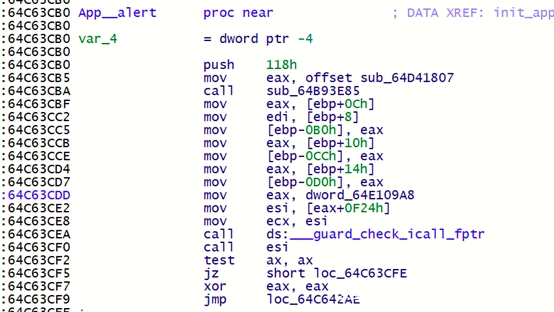Acrobat为了使得EScript可以调用其他模块(动态链接库)实现的Native函数，在SpiderMoneky的Native调用机制上实现了自己的机制。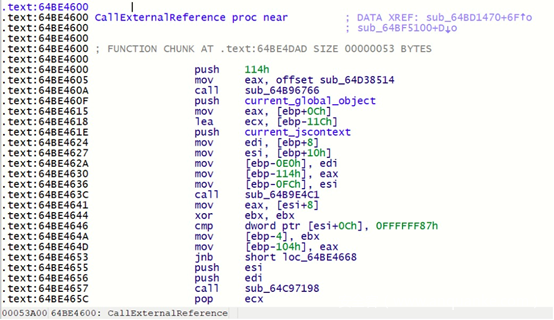app的prototype保存的函数名称对应的Native实现如下。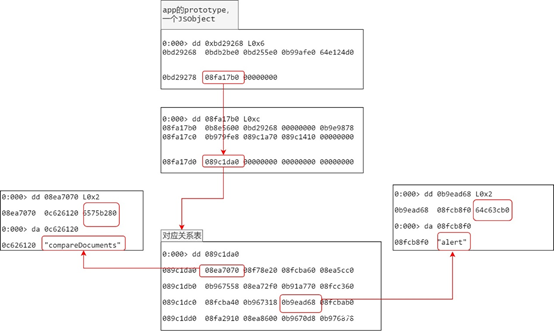# 3 结束语